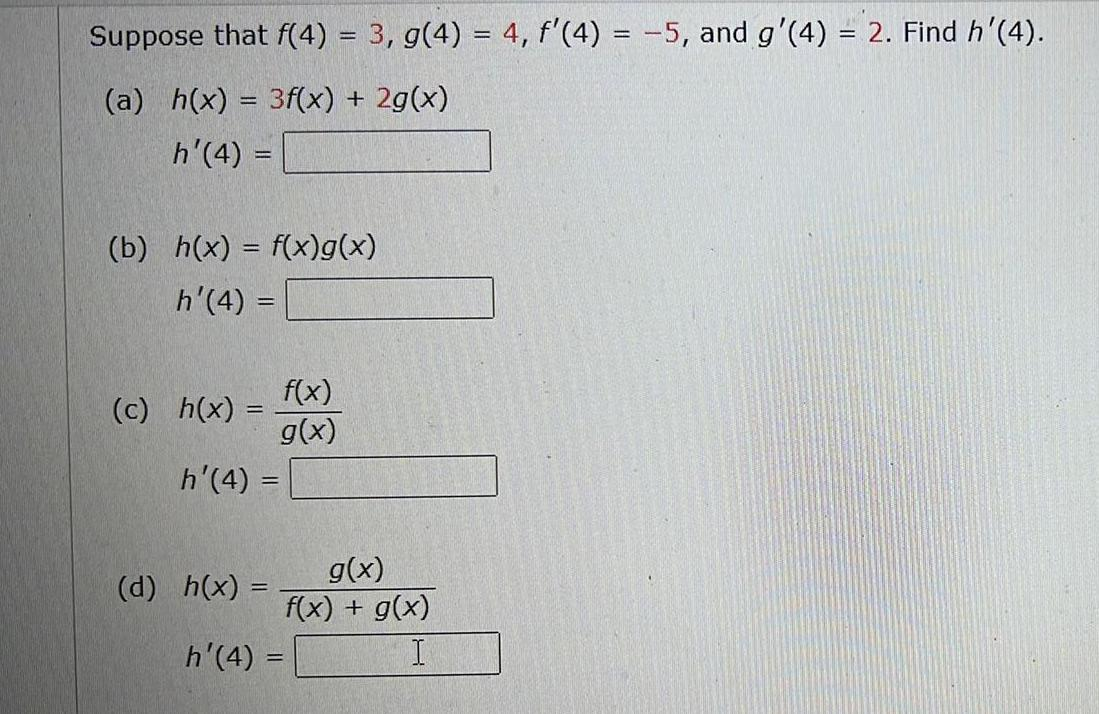Question:

# Suppose that f(4) = 3, g(4) = 4, f'(4) = -5, and g'(4) = 2.

Last updated: 8/14/2022Suppose that f(4) = 3, g(4) = 4, f'(4) = -5, and g'(4) = 2. Find h'(4). (a) h(x) = 3f(x) + 2g(x) h'(4) = (b) h(x) = f(x)g(x) h'(4) = (c) h(x) =f(x)/g(x) h'(4) = (d) h(x) =(g(x))/(f(x) + g(x)) h'(4) =